# Test: Electrical Machines- 2

## 10 Questions MCQ Test GATE Electrical Engineering (EE) 2023 Mock Test Series | Test: Electrical Machines- 2

Description
Attempt Test: Electrical Machines- 2 | 10 questions in 30 minutes | Mock test for Electrical Engineering (EE) preparation | Free important questions MCQ to study GATE Electrical Engineering (EE) 2023 Mock Test Series for Electrical Engineering (EE) Exam | Download free PDF with solutions
*Answer can only contain numeric values
QUESTION: 1

### A 110/220 V, 60 Hz, single phase, 1-kVA transformer has leakage reactance of 4%. Calculate its total leakage inductance (in mH) referred to 110 V side.

Solution:

Assuming the 110-V side 1 and the 220-V side to be side 2, N1/N2 = 0.5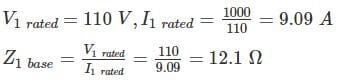Total leakage reactance referred to side 1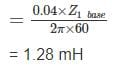QUESTION: 2

### A single phase transformer has 350 and 700 turns in its primary and secondary winding. The primary is connected to a 400 V, 50 Hz ac supply. If the net cross sectional area of core is 50 cm2, the maximum value of flux density in the core is

Solution:

E1 = 4.44 BmNAf
⇒ 400 = 4.44 × Bm × 50 × 10−4 × 50 × 350
⇒ Bm = 1.03T

*Answer can only contain numeric values
QUESTION: 3

### A single phase transformer takes a no load current of 1.3 A when high voltage winding is kept open. If the iron loss component of no load current is 0.5 A, the magnetizing component of no load current will be____ (in A)

Solution:

No load current I0 = 1.3 A
Iron loss component IW = 0.5 A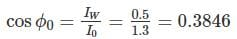Magnetizing component of no load current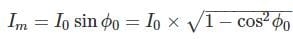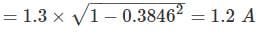QUESTION: 4

For a specified input voltage and frequency, if the equivalent radius of the core of a transformer is reduced by half, the factor by which the number of turns in the primary should change to maintain the same no load current is

Solution:

EMT equation of Transformer is given by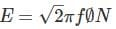Where f is the flux density, given as ϕ = B × A
Now, the given radius is reduced by half, i.e.  r → r/2,
So, the area will be reduced by 1/4 i.e. to maintain no load current constant (in turn emf (EE)) to be constant, number of turns will be 4 times

*Answer can only contain numeric values
QUESTION: 5

The maximum efficiency of a 100 kVA, signal phase transformer is 98% and occurs at 80% of full load at 0.8 P.F. If the leakage impedance of the transformer is 5%, find voltage regulation (in %) at rated load of 0.8 power factor lagging.

Solution:

Since maximum efficiency occurs at 80% of full load at 0.8 P.F
Output at ηmax = (100 × 0.8) × 0.8 = 64 kW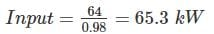∴ Total loss = 65.3 – 64 = 1.3 kW
This loss is divided equally between cu and iron
∴ Cu loss at 80% of full load = 1.3/2 = 0.65 kW
Cu loss at full load = 0.65/82 = 1 kW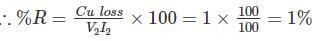∴ % Regulation = [1 × 0.8 + 5 × 0.6] = 3.8%

*Answer can only contain numeric values
QUESTION: 6

A 600 kVA transformer has an efficiency of 95% at full load and also at 60% of full load; both at U.P.F. Determine the efficiency of transformer at 3/4 th full load.

Solution: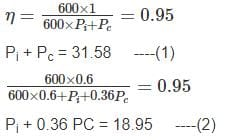Salving equs (1) and (2) we get
Pi = 11.846
Pc = 19.734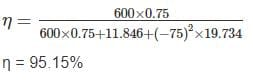*Answer can only contain numeric values
QUESTION: 7

A 220/115 V, 25 Hz single phase transformer has eddy current loss 150W, which is half of the no load loss at rated applied voltage. If the transformer is used with primary 50 Hz 440 V, the total no load loss would be

Solution:

Eddy current loss = 150 W
No load loss W0 = 300 W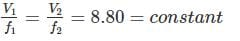Now W0 = Wh + We
300 = Wh + 150
W= 150 W
Wh ∝ f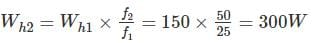Wh ∝ f2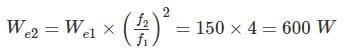∴ Total no load loss = We2 + Wh2 = 600 + 300 = 900 W

*Answer can only contain numeric values
QUESTION: 8

The no-load voltage of the transformer is 440V and It's load current 10A. If the equivalent resistance, Reactance is 0.12Ω and 2.42Ω and is operating at a power factor of 0.8 lag, then the voltage Regulation of transformer is _________%

Solution:

P.F. = Cas-1­ 0.8 = 36.86°
V2 = E2 – I∠-ϕ (R2 + jX2) = 440 – 10∠-36.86 (0.12 + j2.42)
= 424.93 ∠-2.51° V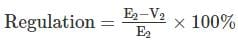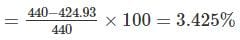QUESTION: 9

A 1 kVA, 200/100 V, 50 Hz, single phase transformer gave the following results on 50 Hz:
OC (LV side): 100 V, 20 watts
SC (HV side): 5 A, 25 watts
It is assumed that no load loss components are equally divided. The above tests were then conducted on the same transformer at 40 Hz. Test results are
OC (HV): 160 V, W watts
SC (LV): 10 A, W watts
Neglecting skin effect, W and Wwill be

Solution:

Given that no load losses components are equally divided
Wh = We = 10W
Initially test is conducted on LV side
Now V/f ratio is 100/50 = 2
In HV side, applied voltage is 160 V, this voltage on LV side is equal to 80 V.
Now V/f ratio is constant, Wh ∝ f, We ∝ f2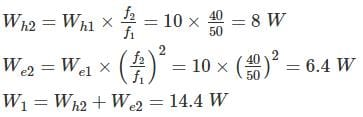In SC test
I(HV side) = 5 A
I(LV side) = 10 A
As the SC tests were conducted at rated current on both sides, the copper losses are same.

*Answer can only contain numeric values
QUESTION: 10

A 50 Hz, single phase transformer draws a short circuit current of 30 A at 0.2 power factor lag when connected to 16V, 50 Hz source. The short circuit current when the same transformer is energized from 16V, 25 Hz source is____ (in A)

Solution: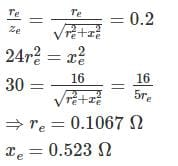At 25 Hz, xe = 0.2615Ω, re = 0.1067Ω
⇒ ze = 0.2822Ω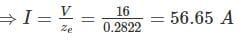Use Code STAYHOME200 and get INR 200 additional OFF Use Coupon Code